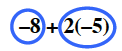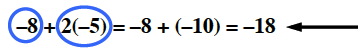### Home > MC1 > Chapter 2 > Lesson 2.3.2 > Problem2-118

2-118.

Copy each expression below and circle each term. Then simplify each expression.

1.  $−8+2(−5)$

Begin by circling each term.Simply the expression.

$−18$

1.  $3(7+2)+4$

Follow the steps outlined in part (a).

$31$. Remember to circle each term!

1.  $4(−2+1+7)$

Follow the steps outlined in part (a).

1.  $5(6+2)+4+2(−5+8)$

Follow the steps outlined in part (a).